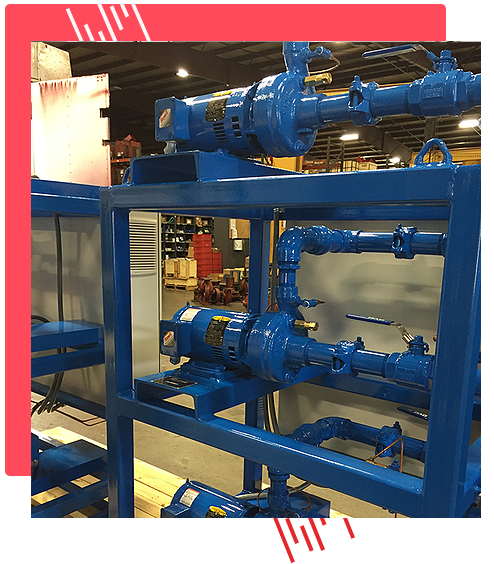ELBIFor tank diameters 14” or less the A (in) is 12.69, B (in) is 11.00, C (in) is 1.19 and D (npt) is ½. For 20” A (in) is 18.19, B (in) is 16.50, C (in) is 1.19 and D (npt) ½. For 24” A (in) is 24.44, B (in) is 22.75, C (in) is 1.19 and D (npt) is ½. For 30” A (in) is 29.94, B (in) is 28.25, C (in) is 1.19 and D (npt) is ½.

VIEW DOCUMENTATION

## PRODUCTS REPRESENTED